# SQL Server Variable: Declare, Set, Select, Global,Local [TSQL Examples]

## What is Variable?

In MS SQL, variables are the object which acts as a placeholder to a memory location. Variable hold single data value.

In this tutorial you will learn:

## Types of Variable: Local, Global

MS SQL has two types of variables:

1. Local variable
2. Global variable.

However, the user can only create a local variable.

Below figure explain two types of variable available in MS SQL server.

### Local variable:

• A user declares the local variable.
• By default, a local variable starts with @.
• Every local variable scope has the restriction to the current batch or procedure within any given session.

### Global variable:

• The system maintains the global variableA user cannot declare them.
• The global variable starts with @@
• It stores session related information.

## How to DECLARE a variable

• Before using any variable in batch or procedure, you need to declare the variable.
• DECLARE command is used to DECLARE variable which acts as a placeholder for the memory location.
• Only once the declaration is made, a variable can be used in the subsequent part of batch or procedure.

TSQL Syntax:

`DECLARE  { @LOCAL_VARIABLE[AS] data_type  [ = value ] } `

Rules:

• Initialization is an optional thing while declaring.
• By default, DECLARE initializes variable to NULL.
• Using the keyword ‘AS’ is optional.
• To declare more than one local variable, use a comma after the first local variable definition, and then define the next local variable name and data type.

### Examples of Declaring a variable:

Query: With ‘AS’

```DECLARE @COURSE_ID AS INT;
```

Query: Without ‘AS’

```DECLARE @COURSE_NAME VARCHAR (10);
```

Query: DECLARE two variables

```DECLARE @COURSE_ID AS INT, @COURSE_NAME VARCHAR (10);
```

## Assigning a value to a VARIABLE

You can assign a value to a variable in the following three ways:

1. During variable declaration using DECLARE keyword.
2. Using SET
3. Using SELECT

Let’s have a look at all three ways in detail:

### During variable declaration using DECLARE keyword.

T-SQL Syntax:

`DECLARE { @Local_Variable [AS] Datatype [ = value ] }`

Here, after datatype we can use ‘=’ followed by value to be assigned

Query:

```DECLARE @COURSE_ID AS INT = 5
PRINT @COURSE_ID
```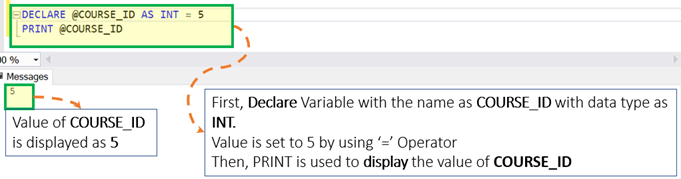### Using SET

Sometimes we want to keep declaration and initialization separate. SET can be used to assign values to the variable, post declaring a variable.Below are the different ways to assign values using SET:

Example: Assigning a value to a variable using SET

Syntax:

```DECLARE @Local_Variable <Data_Type>
SET @Local_Variable =  <Value>
```

Query:

```DECLARE @COURSE_ID AS INT
SET @COURSE_ID = 5
PRINT @COURSE_ID
```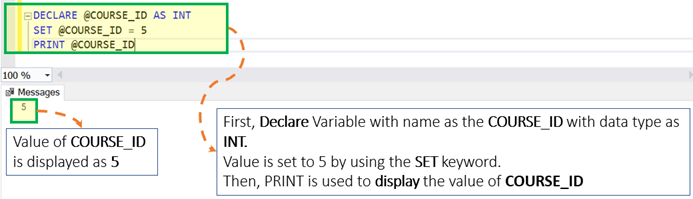Example: Assign a value to multiple variables using SET.

Syntax:

```DECLARE @Local_Variable _1 <Data_Type>, @Local_Variable_2 <Data_Type>,
SET @Local_Variable_1 = <Value_1>
SET @Local_Variable_2 = <Value_2>```

Rule: One SET Keyword can be used to assign a value to only one variable.

Query:

```DECLARE @COURSE_ID as INT, @COURSE_NAME AS VARCHAR(5)
SET @COURSE_ID = 5
SET @COURSE_NAME = 'UNIX'
PRINT @COURSE_ID
PRINT @COURSE_NAME
```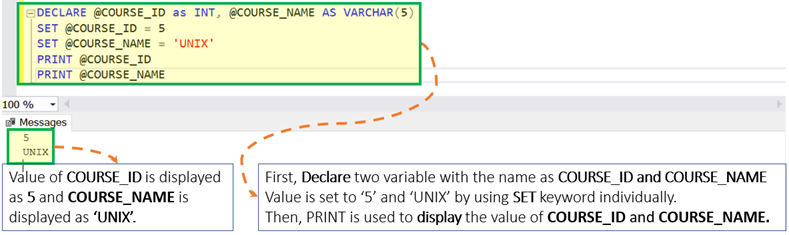Example: Assigning a value to a variable with a Scalar Subquery using SET

Syntax:

`DECLARE @Local_Variable_1 <Data_Type>, @Local_Variable_2 <Data_Type>,SET @Local_Variable_1 = (SELECT <Column_1> from <Table_Name> where <Condition_1>)`

Rules:

• Enclose the query in parenthesis.
• The query should be a scalar query. A scalar query is a query with results as just one row and one column. Otherwise, the query will throw an error.
• If the query returns zero rows, then the variable is set to EMPTY, i.e., NULL.

Assumption: Assume that we have the table as ‘Guru99’ with two columns as displayed below: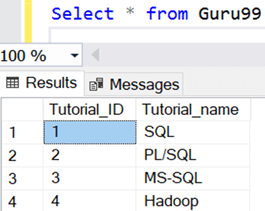We will use ‘Guru99’ table in the further tutorials

Example 1: When subquery return one row as a result.

```DECLARE @COURSE_NAME VARCHAR (10)
SET @COURSE_NAME = (select Tutorial_name from Guru99 where Tutorial_ID = 3)
PRINT @COURSE_NAME
```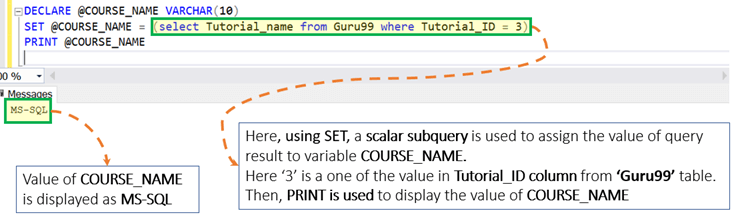Example 2: When subquery returns zero row as a result

```DECLARE @COURSE_NAME VARCHAR (10)
SET @COURSE_NAME = (select Tutorial_name from Guru99 where Tutorial_ID = 5)
PRINT @COURSE_NAME
```

In this particular case, the variable value is EMPTY, i.e., NULL.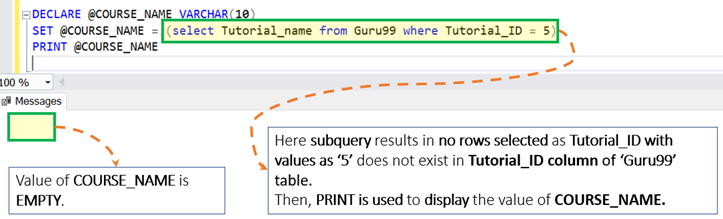### USING SELECT

Just like SET, we can also use SELECT to assign values to the variables, post declaring a variable using DECLARE. Below are different ways to assign a value using SELECT:

Example: Assigning a value to a variable using SELECT

Syntax:

```DECLARE @LOCAL_VARIABLE <Data_Type>
SELECT @LOCAL_VARIABLE = <Value>```

Query:

```DECLARE @COURSE_ID INT
SELECT @COURSE_ID = 5
PRINT @COURSE_ID
```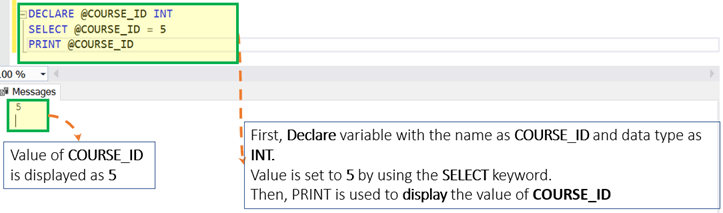Example: Assigning a value to multiple variable using SELECT

Syntax:

.

`DECLARE @Local_Variable _1 <Data_Type>, @Local_Variable _2 <Data_Type>,SELECT @Local_Variable _1 = <Value_1>,  @Local_Variable _2 = <Value_2>`

Rules: Unlike SET, SELECT can be used to assign a value to multiple variables separated by the comma.

```DECLARE @COURSE_ID as INT, @COURSE_NAME AS VARCHAR(5)
SELECT @COURSE_ID = 5, @COURSE_NAME = 'UNIX'
PRINT @COURSE_ID
PRINT @COURSE_NAME
```Example: Assigning the value to a variable with a Subquery using SELECT

Syntax:

`DECLARE @Local_Variable_1 <Data_Type>, @Local_Variable _2 <Data_Type>,SELECT @Local_Variable _1 = (SELECT <Column_1> from <Table_name> where <Condition_1>)`

Rules:

• Enclose the query in Parenthesis.
• The query should be a scalar query. The scalar query is the query with the result as one row and one column. Otherwise, the query will throw an error.
• If the query returns zero rows, then the variable is EMPTY, i.e., NULL.
• Reconsider our ‘Guru99’ table

Example 1: When subquery return one row as a result.

```DECLARE @COURSE_NAME VARCHAR (10)
SELECT @COURSE_NAME = (select Tutorial_name from Guru99 where Tutorial_ID = 1)
PRINT @COURSE_NAME
```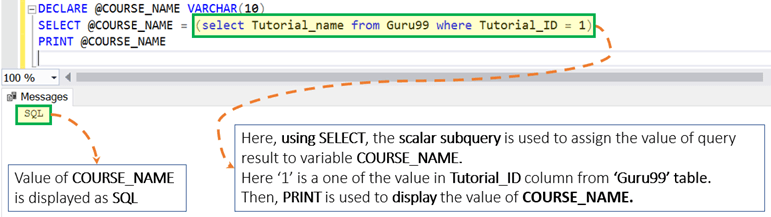Example 2: When subquery return zero row as a result

```DECLARE @COURSE_NAME VARCHAR (10)
SELECT @COURSE_NAME = (select Tutorial_name from Guru99 where Tutorial_ID = 5)
PRINT @COURSE_NAME
```

In this particular case, the variable is to EMPTY, i.e., NULL.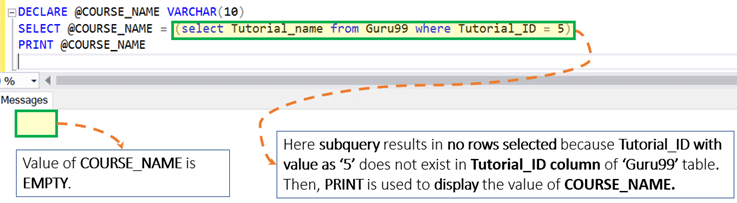Example 3: Assign a value to a variable with a regular SELECT statement.

Syntax:

`DECLARE @Local_Variable _1 <Data_Type>, @Local_Variable _2 <Data_Type>,SELECT @Local_Variable _1 = <Column_1> from <Table_name> where <Condition_1>`

Rules:

• Unlike SET, if the query results in multiple rows then the variable value is set to the value of the last row.
• If the query returns zero rows, then the variable is set to EMPTY, i.e., NULL.

Query 1: The query returns one row.

```DECLARE @COURSE_NAME VARCHAR (10)
SELECT @COURSE_NAME = Tutorial_name from Guru99 where Tutorial_ID = 3
PRINT @COURSE_NAME
```Query 2: The query returns multiple rows.

```DECLARE @COURSE_NAME VARCHAR (10)
SELECT @COURSE_NAME = Tutorial_name from Guru99
PRINT @COURSE_NAME
```

In this special case, variable value is set to the value of the last row.Query 3: The query returns zero rows.

```DECLARE @COURSE_NAME VARCHAR (10)
SELECT @COURSE_NAME = Tutorial_name from Guru99 where Tutorial_ID = 5
PRINT @COURSE_NAME
```

In this particular case, the variable is EMPTY, i.e., NULL.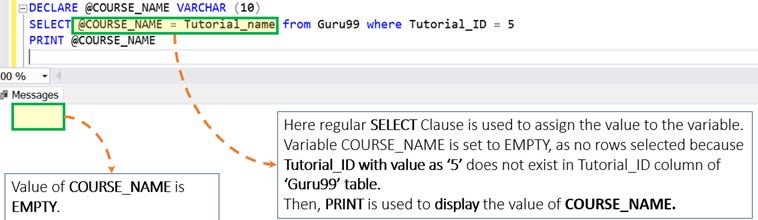### Other Examples

Using variable in the query

Query:

```DECLARE @COURSE_ID Int = 1
SELECT * from Guru99 where Tutorial_id = @COURSE_ID
```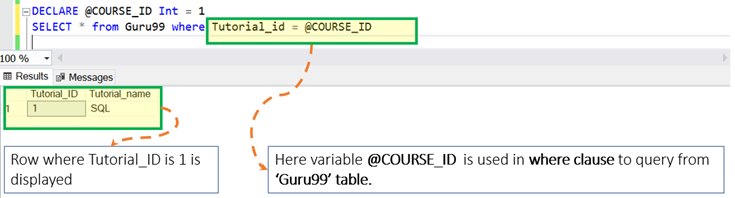## Interesting Facts!

• A local variable can be displayed using PRINT as well as SELECT COMMAND
• Table Data type doesn’t allow the use of ‘AS’ during declaration.
• SET complies with ANSI standards whereas SELECT does not.
• Creating a local variable with the name as @ is also allowed. We can declare it as, for example:
```'DECLARE @@ as VARCHAR (10)'
```

## Summary:

• Variables are the object which acts as a placeholder.
• Two types of Variable exist: Local and Global
• We can assign the variable in the following three ways:

While using DECLARE
Using SET
USING SELECT

.